# rigid-body dynamics

(redirected from Rigid body dynamics)
Also found in: Acronyms, Wikipedia.

## Rigid-body dynamics

The study of the motion of a rigid body under the influence of forces. A rigid body is a system of particles whose distances from one another are fixed. The general motion of a rigid body consists of a combination of translations (parallel motion of all particles in the body) and rotations (circular motion of all particles in the body about an axis). Its equations of motion can be derived from the equations of motion of its constituent particles. See Rectilinear motion, Rotational motion

The location of a mass point mi can be specified relative to a fixed-coordinate system by a position vector with cartesian components (xi,yi,zi). The vector force which acts on the mass points has corresponding components (Fix,Fiy,Fiz). Newton's second law for the motion of mi is stated in Eq. (1).

(1)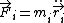Here ≡ d2i/dt2 = is the acceleration of mi. See Acceleration, Force, Newton's laws of motion

#### Translational motion

If Eq. (1) is summed over all particles in the rigid body, the left-hand side becomes the total force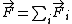acting on the rigid body. If the internal forces satisfy Newton's third law (to each action there is an equal but opposite reaction), the contributions of the internal forces cancel in pairs and is the total external force on the rigid body, ext. The right-hand side can be expressed in terms of the center-of-mass (CM) position vector defined by Eq. (2),

(2)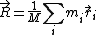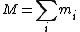where M is the total mass of the body. Then the sum of the equations of motion, Eq. (1), takes the form of Eq. (3). This equation
(3)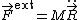of motion for the center of mass of the rigid body has exactly the form of the equation of motion for a particle of mass M and position , under the influence of an external force ext. Consequently, the second law of motion holds, not just for a particle, but for an arbitrary rigid body, if the position of the body is interpreted to mean the position of its center of mass. See Center of mass

The momentum of a mass point is given by the product of the mass and the velocity, i = mi, where ≡. The total momentum of the center of mass of the rigid body, obtained by summing over the momenta of its constituent masses, is given by Eq. (4). In terms of the center-of-mass momentum , the equation of motion for the center of mass is expressed by Eq. (5).

(4)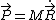(5)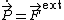For an isolated rigid body, the external force is zero and therefore is constant. According to Eq. (4), this implies that the center of mass moves with constant velocity = . See Conservation of momentum, Momentum

In fact, the preceding equations for translational motion hold for any body, rigid or nonrigid.

#### Rotational motion

The total angular momentum of a rigid body about a point O with coordinate is the sum of the angular momenta of its constituent masses, and is given by Eq. (6).

(6)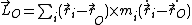Here × denotes the cross-product of the coordinate vector (i - ) with the momentum vector mi(). The time derivative of is given in
(7)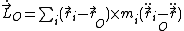Eq. (7). Hereafter the point O is taken to be either a fixed point (in which case ) or the center-of-mass point. Using the equation of motion (1), mii can be replaced by i. Thus the rotational equation of motion (8)
(8)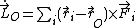is obtained. The right-hand side of Eq. (8) is known as the torque, . The contribution of the internal forces to the torque vanishes if the “extended third law” holds; namely, action equals reaction and is directed along a line between the particles. In this circumstance the rotational equation of motion is given by Eqs. (9),
(9{\it a})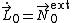(9{\it b})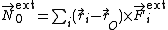where ext is the total torque associated with external forces that act on the rigid body. See Angular momentum, Torque

It is straightforward to show from Eq. (6) that the angular momentum about an arbitrary point O is related to the angular momentum about the center of mass by Eq. (10). The torque about an arbitrary point O can also be easily related to the torque about the center of mass by Eq. (11).

(10)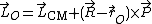(11)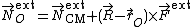Six coordinates determine the positions of all particles in a rigid body, and the motion of a rigid body is described by six equations of motion. The translational motion of the center of mass is determined by Eq. (5), and the rotational motion about the center of mass, or a fixed point, is determined by Eq. (9). These six equations, which hold for any system of particles, completely describe the motion of a rigid body.

#### Motion of an isolated system

The equation = ext has the same form as = ext. Both and are constants for an isolated system since ext = 0 and ext = 0. Even though the two conditions ext = 0 and ext = 0 appear similar, there are some important differences for systems in which internal motion is possible. If ext = 0, a center of mass which is at rest will remain so, regardless of internal forces or internal motion. If ext = 0, the total angular momentum is constant, and if initially zero, will remain zero. However, = 0 does not exclude changes in orientation of the system by the use of merely internal forces. There is no rotational analog to the equation (t) = + (0) for linear motion of the center of mass.

#### Static equilibrium

In the design of permanent structures, the conditions under which a rigid body remains in steady motion under the action of a set of forces are of great importance. The six conditions for complete equilibrium of a rigid body are given in Eqs. (12). However, in many

(12)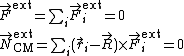circumstances equilibrium is desired only for a subset of the six independent directions of motion. To illustrate, the external force in the direction of motion of an accelerating automobile is nonzero, but equilibrium is maintained in all other directions. See Statics

## rigid-body dynamics

[′rij·id ¦bäd·ē dī′nam·iks]
(mechanics)
The study of the motions of a rigid body under the influence of forces and torques.
Site: Follow: Share:
Open / Close